# Question 4 Given that f(x) = X-4 (x + 1)(x-3) Which of the following statement is...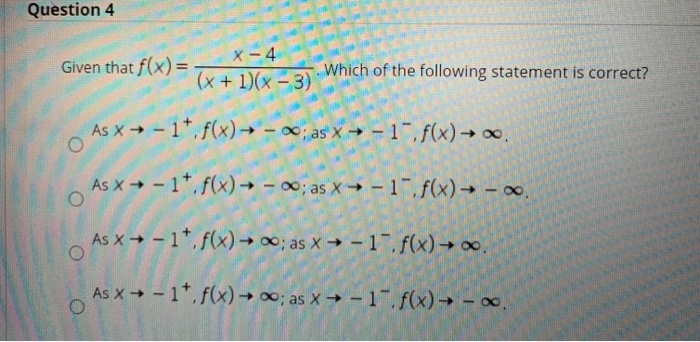Question 4 Given that f(x) = X-4 (x + 1)(x-3) Which of the following statement is correct? As x + -1*. f(x) — - ~; as x + -1 f(x) → . As x + - 1+ f(x) → - 20; as x → -1-f(x) — - As x + -17 f(x) → 0; as x + -1-.f(x) → 0. As X - - 1* f(x) → 0; as X →-1-f(x) - 0.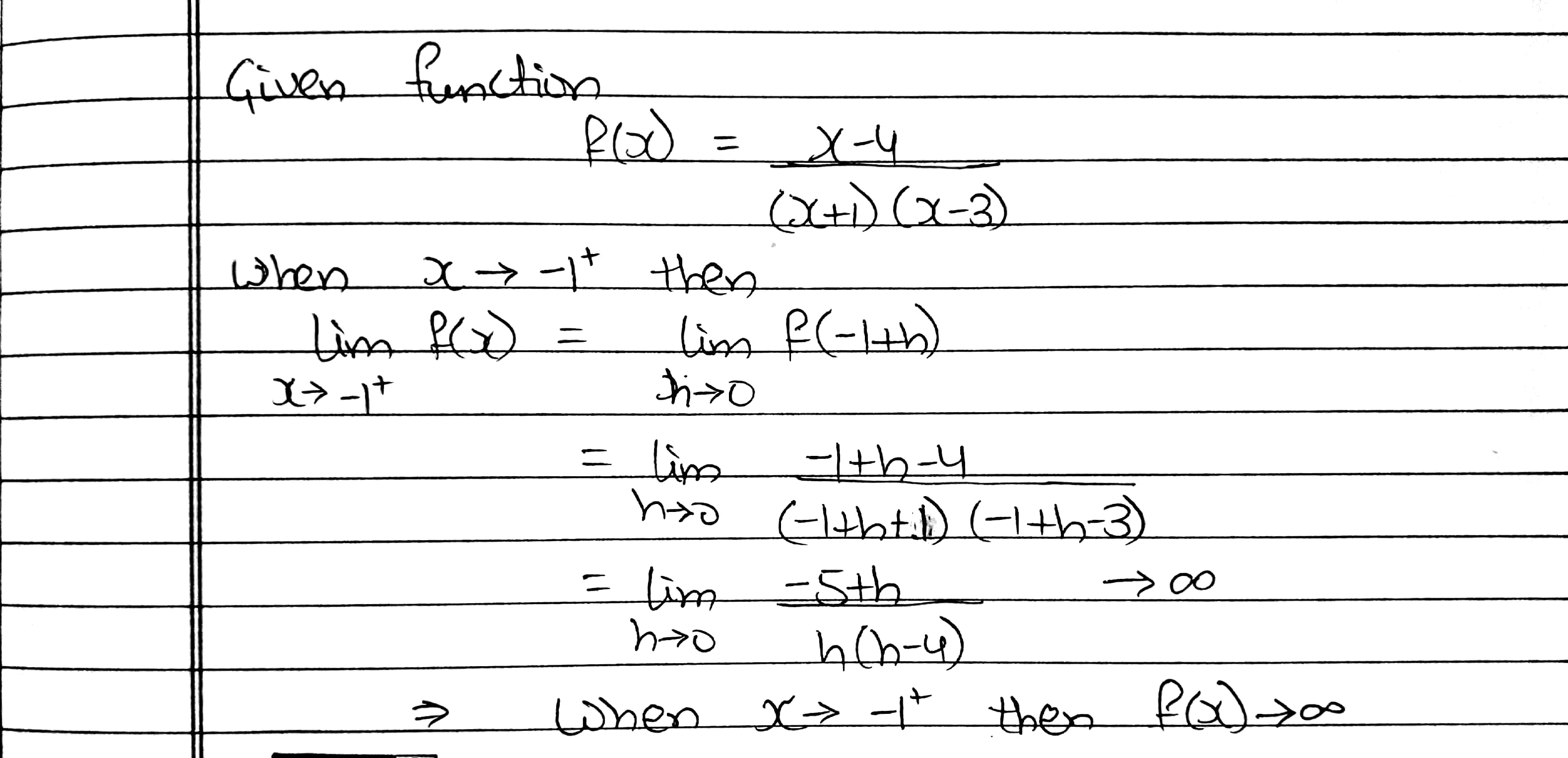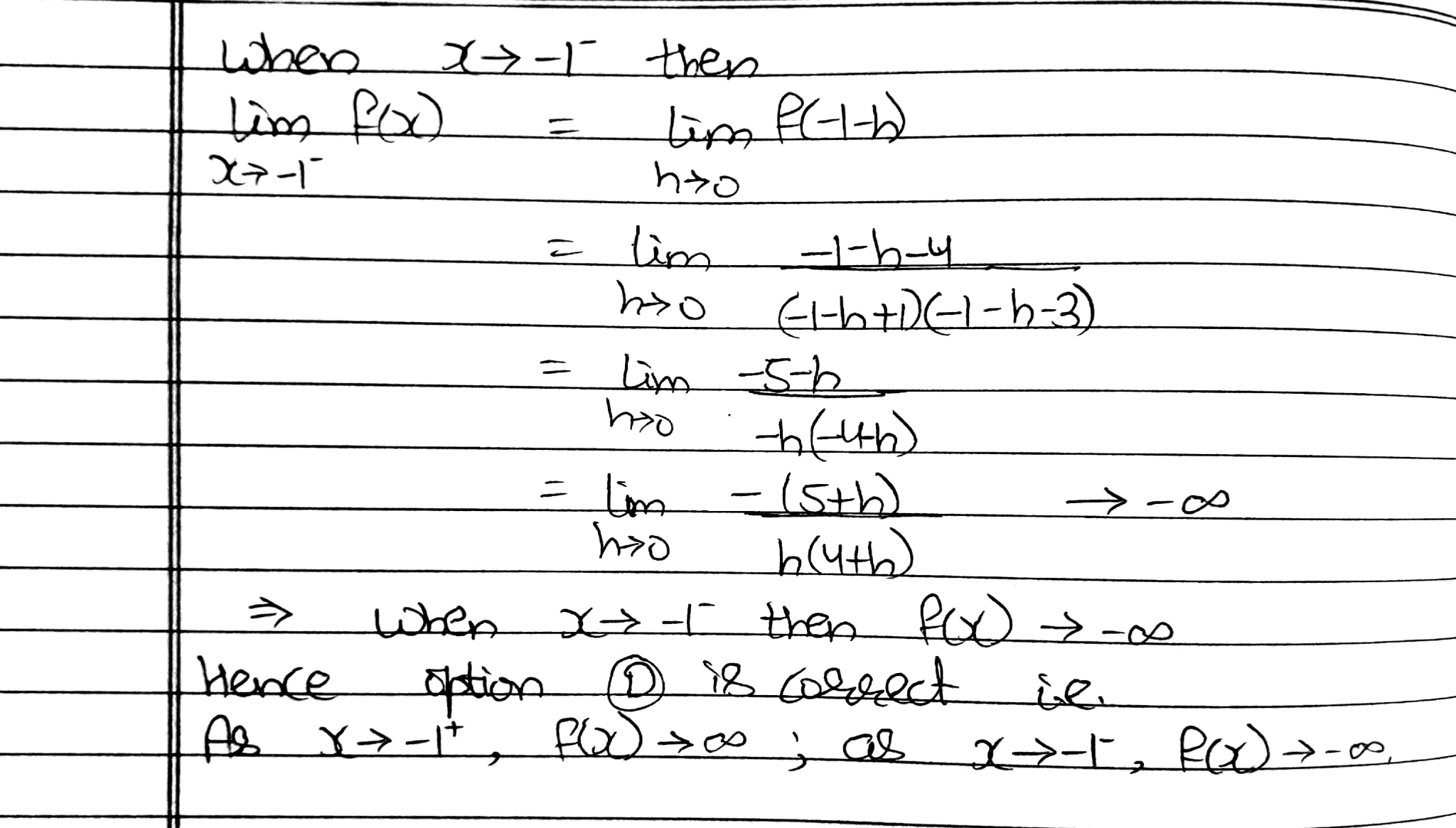PLEASE RATE IF IT WAS HELPFUL FOR YOU.

##### Add Answer of: Question 4 Given that f(x) = X-4 (x + 1)(x-3) Which of the following statement is...
Similar Homework Help Questions
• ### Question 4 Given that f(x)= x - 4 (x + 1)(x-3) Which of the following statement...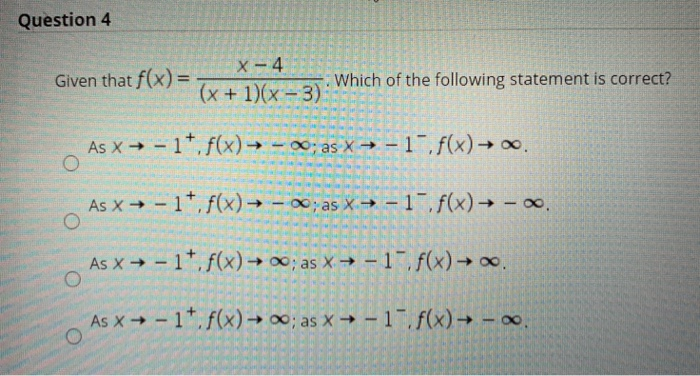Question 4 Given that f(x)= x - 4 (x + 1)(x-3) Which of the following statement is correct? As x → - 17.f(x) — - 20; as X = -1-f(x) → .. As x + - 1*. f(x) — - ~; as x = -1 f(x) - . o As x + - 17. f(x) → 0; as x + -1 f(x) → As → - 17. f(x) → 0; as x + -1 . f(x) - 0. O Question...

• ### Question 5 Given that f(x) = 2x5 – 4x+6 x²-3 Which of the following statement is...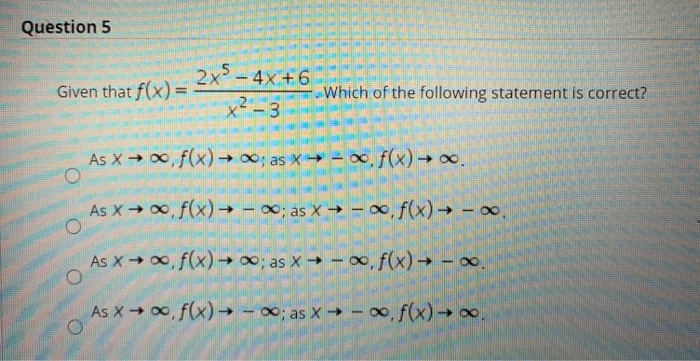Question 5 Given that f(x) = 2x5 – 4x+6 x²-3 Which of the following statement is correct? As X → ,f(x) → 0; as → = f(x) = . As x + f(x) = - *; as X — - ~.f(x) — - ~. O As →, f(x) = 0; as * — - ~,f(x) — -. As x + f(x) → - ; as → - .f(x) – ..

• ### Question 3 Let f(x)be the function of period 4 which is given on the interval (-2,...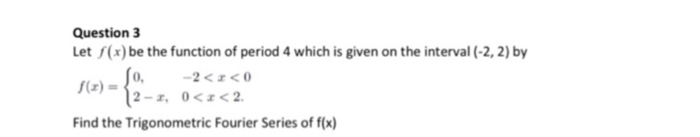Question 3 Let f(x)be the function of period 4 which is given on the interval (-2, 2) by f(z) = Find the Trigonometric Fourier Series of f(x) 0.2<0

• ### 17. Given the function f(x) = ? answer the following questions: [4 Points] x+1 (a) What...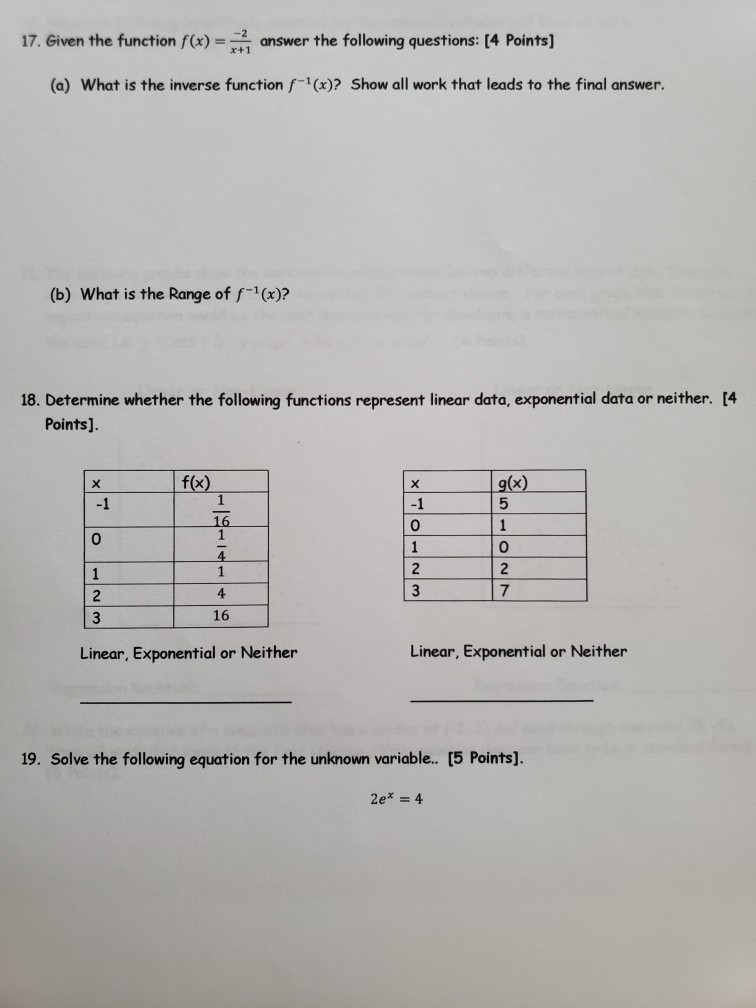17. Given the function f(x) = ? answer the following questions: [4 Points] x+1 (a) What is the inverse function f-(x)? Show all work that leads to the final answer. (b) What is the Range of f-1(x)? 18. Determine whether the following functions represent linear data, exponential data or neither. [4 Points). f(x) х -1 g(x) 5 1 0 16 1 4 1 4 Х -1 0 1 2 3 o 2 7 1 2 3 16 Linear, Exponential or...

• ### (3.2) Consider the data given in the following table 05 1 15 f(x) 0 2 0 6 1 2 20 (4) (a) Approximate f with a functi...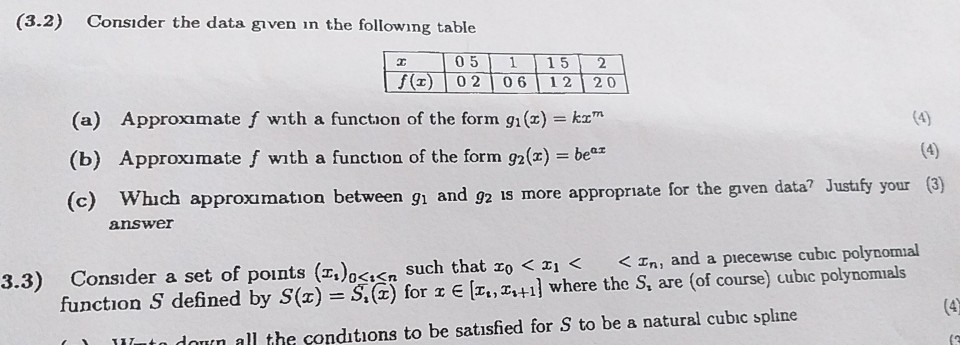(3.2) Consider the data given in the following table 05 1 15 f(x) 0 2 0 6 1 2 20 (4) (a) Approximate f with a function of the form q (x) = kxm (4) (b) Approximate f with a function of the form g2(x) = be Which approximation between q and g2 1s more appropriate for the given data? Justify your (3) (c) answer < In, and a piecewise cubic polynomial Consider a set of points (I,) Such that...

• ### Given f(x)=-x^3+x^2+3x+4, find f(-1), f(0), and f(1)

Given f(x)=-x^3+x^2+3x+4, find f(-1), f(0), and f(1).

• ### please answer this multiple choice question The following statement concerns questions 1-3. The function f(t) is...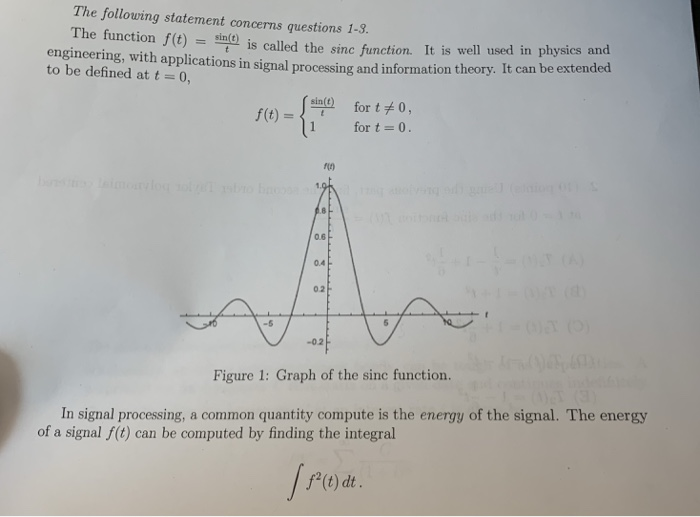please answer this multiple choice question The following statement concerns questions 1-3. The function f(t) is called the sinc function. It is well used in physics and engineering, with applications in signal processing and information theory. It can be extended to be defined at t = 0, sin(t) for t 0 f(t) for t 0. 1.0 0.6 CA 0.4 0.2 -5 -02 Figure 1: Graph of the sinc function. a common quantity compute is the energy of the signal. The...

• ### 3. Given that lim f(x) = 4 and lim f(x) = -4, what is true about...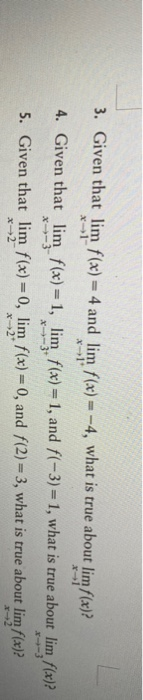3. Given that lim f(x) = 4 and lim f(x) = -4, what is true about lim f(x)? x-1 4. Given that lim f(x) = 1, lim f(x) = 1, and f(-3) = 1, what is true about lim f(x)? 5. Given that lim f(x) = 0, lim f(x) = 0, and f(2) = 3, what is true about limf(x)? X-3 X-3 X-2

• ### (1 point) Given that a function f(x) has a tangent line at x = 4 given...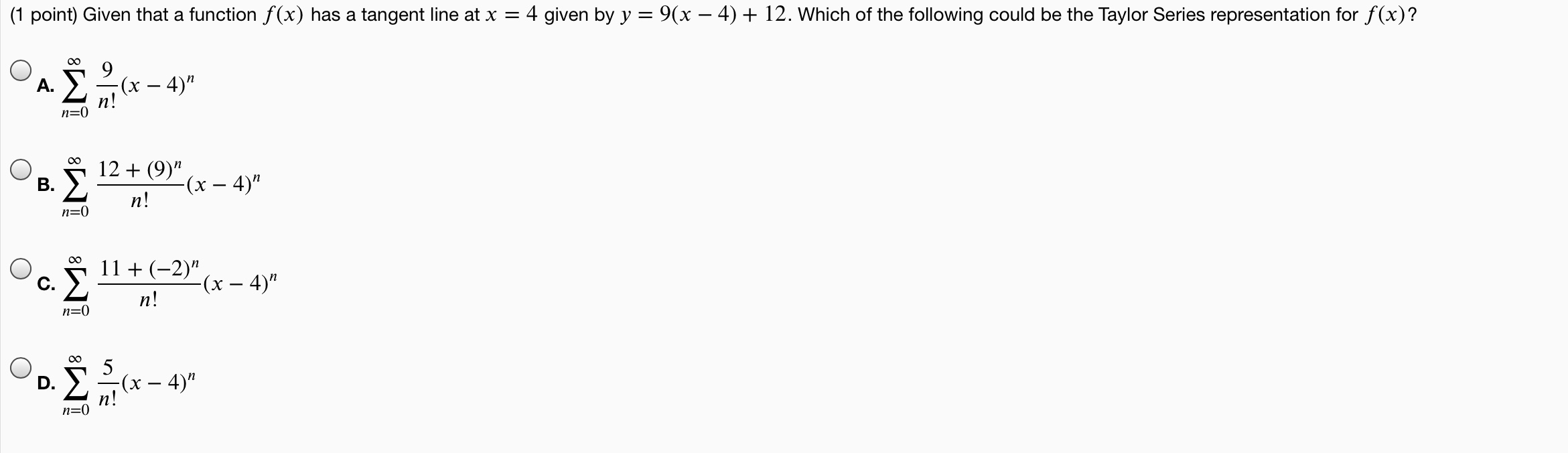(1 point) Given that a function f(x) has a tangent line at x = 4 given by y = 9(x – 4) + 12. Which of the following could be the Taylor Series representation for f(x)? 00 A. (x – 4)" n! n=0 B. 12 + (9)" -(x – 4)" n! n=0 Ž 11 + (-2)" -(x – 4)" n! n=0 -(x – 4)" n! n=0

• ### (1 point) Given that a function f(x) has a tangent line at x = 4 given...(1 point) Given that a function f(x) has a tangent line at x = 4 given by y = 9(x – 4) + 12. Which of the following could be the Taylor Series representation for f(x)? 00 A. (x – 4)" n! n=0 B. 12 + (9)" -(x – 4)" n! n=0 Ž 11 + (-2)" -(x – 4)" n! n=0 -(x – 4)" n! n=0

Free Homework App